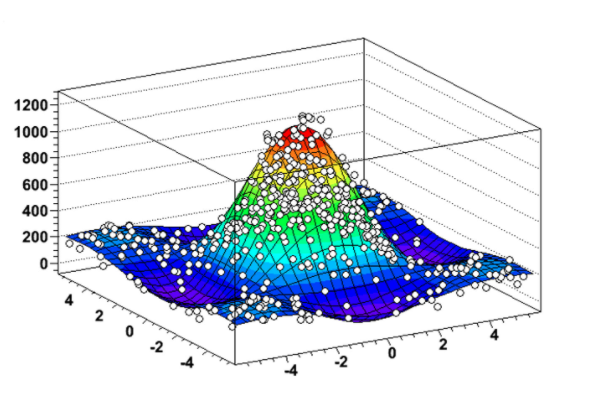# Painting a TEfficiency 3D histogram

Hey experts

I am wondering what do I need to do to be able to fill out a 3D TEfficiency. I tried several things like drawing lego, scatter plots etc but it gives me this error for any draw option:

``````Warning in <TEfficiency::Paint>: Painting 3D efficiency is not implemented
``````

Here is the Make Class macro associated with the TTree:

``````#define pointeff_cxx
#include "pointeff.h"
#include <TH2.h>
#include <TH3.h>
#include <TStyle.h>
#include <TCanvas.h>
#include <TEfficiency.h>
void pointeff::Loop()
{
//   In a ROOT session, you can do:
//      root> .L pointeff.C
//      root> pointeff t
//      root> t.GetEntry(12); // Fill t data members with entry number 12
//      root> t.Show();       // Show values of entry 12
//      root> t.Show(16);     // Read and show values of entry 16
//      root> t.Loop();       // Loop on all entries
//

//     This is the loop skeleton where:
//    jentry is the global entry number in the chain
//    ientry is the entry number in the current Tree
//  Note that the argument to GetEntry must be:
//    jentry for TChain::GetEntry
//    ientry for TTree::GetEntry and TBranch::GetEntry
//
//       To read only selected branches, Insert statements like:
// METHOD1:
//    fChain->SetBranchStatus("*",0);  // disable all branches
//    fChain->SetBranchStatus("branchname",1);  // activate branchname
// METHOD2: replace line
//by  b_branchname->GetEntry(ientry); //read only this branch
//    TH1D* h1 = new TH1D("h1","Denom",100,-M_PI,M_PI);
//    TH1D* h2 = new TH1D("h2","Reconstruction Efficiency v/s Y Angle;Y Angle;Efficiency",100,-M_PI,M_PI);

TH3D* h1 = new TH3D("h1","Denom",100,-50,300,100,-200,200,100,-50,1050);
TH3D* h2 = new TH3D("h2","Reconstruction Efficiency v/s XZ Angle;XZ Angle (radians);Efficiency",100,-50,300,100,-200,200,100,-50,1050);

if (fChain == 0) return;

Long64_t nentries = fChain->GetEntriesFast();

Long64_t nbytes = 0, nb = 0;
for (Long64_t jentry=0; jentry<nentries;jentry++) {
if (ientry < 0) break;
nb = fChain->GetEntry(jentry);   nbytes += nb;
// if (Cut(ientry) < 0) continue;
h1->Fill((muonStartX_det+muonEndX_det/2),(muonStartY_det+muonEndY_det/2),(muonStartZ_det+muonEndZ_det/2));
if(fom_smallest<5)// && tracklength_ratio >0.8)
{
h2->Fill((muonStartX_det+muonEndX_det/2),(muonStartY_det+muonEndY_det/2),(muonStartZ_det+muonEndZ_det/2));
}

}
TEfficiency* eff = new TEfficiency(*h2,*h1);
TCanvas* canvas = new TCanvas("canvas","Hit Info!",1500,500);

//   eff->SetTitle("Efficiency Plot (FOM <5 cm and Tracklength Ratio >0.8);Y C.O.M (cm);Efficiency");
eff->SetTitle("Efficiency Plot (FOM <5 cm) ;X C.O.M (cm);Y C.O.M (cm);Z C.O.M (cm);Efficiency");
//  eff->SetFillColor(kGreen);
eff->Draw();

}

``````

I would appreciate any help. Thanks !

Yes that’s still the case: https://root.cern/doc/master/TEfficiency_8cxx_source.html#l02860
Paint is not implemented in the 3D case.
May be @moneta can comment

Thanks for your reply. So is there no way I can draw 3D TEfficiency ?
Basically what I need to do is make a 3D plot which shows track reconstruction efficiency in different areas of the detector. Something like this:I apologize in advance for my limited knowledge of ROOT.

Any suggestions how I could make this 3D plot?

Thanks !

This plot is not from a 3D histogram but a 2D one drawn with option SURF1.